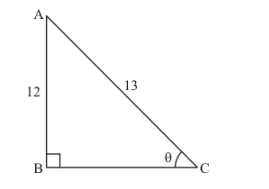# If sin θ=1213, find the value of sin2 θ−cos2 θ2 sin θ cos θ×1tan2 θ.

Question:

If $\sin \theta=\frac{12}{13}$, find the value of $\frac{\sin ^{2} \theta-\cos ^{2} \theta}{2 \sin \theta \cos \theta} \times \frac{1}{\tan ^{2} \theta}$

Solution:

Given: $\sin \theta=\frac{12}{13}$

To Find: The value of expression $\frac{\sin ^{2} \theta-\cos ^{2} \theta}{2 \sin \theta \cos \theta} \times \frac{1}{\tan ^{2} \theta}$

Now, we know that

$\sin \theta=\frac{\text { Perpendicular side opposite to } \angle \theta}{\text { Hypotenuse }}$....(2)

Now when we compare equation (1) and (2)

We get,

Perpendicular side opposite to $\angle \theta=12$

And

Hypotenuse = 13

Therefore, Triangle representing angle $\theta$ is as shown belowBase side BC is unknown and it can be found by using Pythagoras theorem

Therefore by applying Pythagoras theorem

We get,

$A C^{2}=A B^{2}+B C^{2}$

Therefore by substituting the values of known sides

We get,

$13^{2}=12^{2}+B C^{2}$

Therefore,

$B C^{2}=13^{2}-12^{2}$

$B C^{2}=169-144$

$B C^{2}=25$

$B C=\sqrt{25}$

Therefore,

$B C=5 \ldots \ldots(3)$

Now, we know that

$\cos \theta=\frac{\text { Base side adjacent to } \angle \theta}{\text { Hypotenuse }}$

Now from figure (a)

We get,

$\cos \theta=\frac{B C}{A C}$

Therefore from figure (a) and equation (3) ,

$\cos \theta=\frac{5}{13}$....(4)

Now we know that,

$\tan \theta=\frac{\sin \theta}{\cos \theta}$

Therefore, substituting the value of $\sin \theta$ and $\cos \theta$ from equation (1) and (4)

We get,

$\tan \theta=\frac{\frac{12}{13}}{\frac{5}{13}}$

$\tan \theta=\frac{12}{13} \times \frac{15}{5}$

Therefore 13 gets cancelled and we get

$\tan \theta=\frac{12}{5}$...(5)

Now we substitute the value of $\sin \theta, \cos \theta$ and $\tan \theta$ from equation (1), (4) and (5) respectively in the expression below

$\frac{\sin ^{2} \theta-\cos ^{2} \theta}{2 \sin \theta \cos \theta} \times \frac{1}{\tan ^{2} \theta}$

Therefore,

We get,

$\frac{\sin ^{2} \theta-\cos ^{2} \theta}{2 \sin \theta \cos \theta} \times \frac{1}{\tan ^{2} \theta}=\frac{\left(\frac{12}{13}\right)^{2}-\left(\frac{5}{13}\right)^{2}}{2 \times\left(\frac{12}{13}\right) \times\left(\frac{5}{13}\right)} \times \frac{1}{\left(\frac{12}{5}\right)^{2}}$

Therefore by further simplifying we get,

$\frac{\sin ^{2} \theta-\cos ^{2} \theta}{2 \sin \theta \cos \theta} \times \frac{1}{\tan ^{2} \theta}=\frac{\frac{(12)^{2}}{(13)^{2}}-\frac{(5)^{2}}{(13)^{2}}}{2 \times\left(\frac{12}{13}\right) \times\left(\frac{5}{13}\right)} \times \frac{1}{\frac{(12)^{2}}{(5)^{2}}}$

$=\frac{\frac{144}{169}-\frac{25}{169}}{\frac{2 \times 12 \times 5}{13 \times 13}} \times \frac{25}{144}$

$=\frac{\frac{144-25}{169}}{\frac{120}{169}} \times \frac{25}{144}$

$=\frac{119}{169} \times \frac{169}{120} \times \frac{25}{144}$

Now 169 gets cancelled and $\frac{25}{120}$ gets reduced to $\frac{5}{24}$

Therefore

$\frac{\sin ^{2} \theta-\cos ^{2} \theta}{2 \sin \theta \cos \theta} \times \frac{1}{\tan ^{2} \theta}=\frac{119}{1} \times \frac{1}{24} \times \frac{5}{144}$

$=\frac{119 \times 5}{24 \times 144}$

$=\frac{595}{3456}$

Therefore the value of $\frac{\sin ^{2} \theta-\cos ^{2} \theta}{2 \sin \theta \cos \theta} \times \frac{1}{\tan ^{2} \theta}$ is $\frac{595}{3456}$

That is $\frac{\sin ^{2} \theta-\cos ^{2} \theta}{2 \sin \theta \cos \theta} \times \frac{1}{\tan ^{2} \theta}=\frac{595}{3456}$